Function Repository Resource:

# BranchialHypergraph

Compute the branchial hypergraph of a graph

Contributed by: Nikolay Murzin
 ResourceFunction["BranchialHypergraph"][g] computes the branchial hypergraph of a graph g. ResourceFunction["BranchialHypergraph"][g,n] computes the branchial hypergraph of a graph g up to the nth level of ancestry.

## Details and Options

A branchial hypergraph consists of ordered hyperedges encoding the ancestry degree of separation between an initial vertex and other vertices. For example, hyperedge {a,b,c,d} means that vertices a and b have an immediate common ancestor, a and c have a common ancestor of degree 2 (an ancestor at maximum distance of 2 from both a and c), and vertices a and d have a common ancestor of degree 3.
ResourceFunction["BranchialHypergraph"] includes the following option:
 "IncludeUnary" False whether to include unary hyperedges

## Examples

### Basic Examples (4)

Compute branchial hyperedges of a simple graph:

 In:=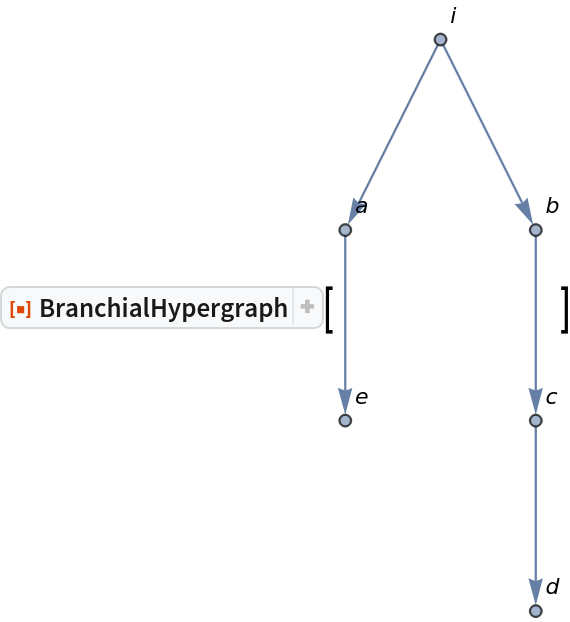Out=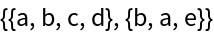Branchial hypergraph of a mixed graph:

 In:=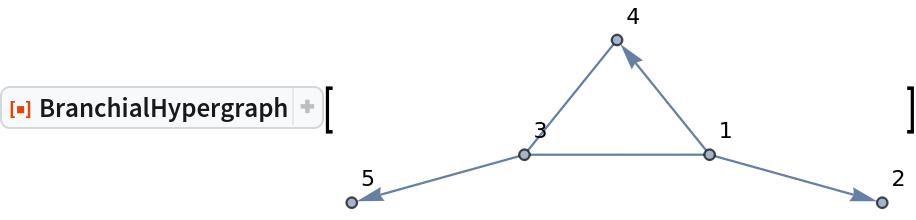Out=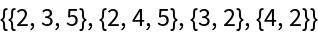Specify the maximum level of ancestry:

 In:=Out=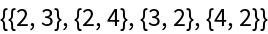The branchial hypergraph of any undirected graph is empty:

 In:=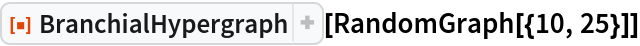Out=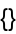### Scope (2)

Generate a simple string substitution multiway system with the resource function MultiwaySystem and compute its branchial hypergraph:

 In:=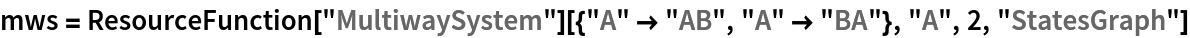Out=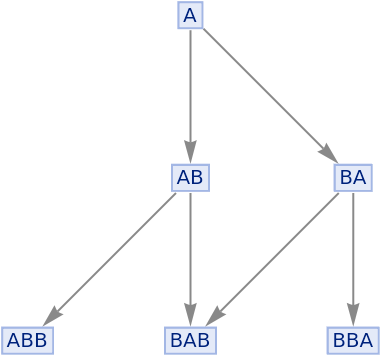In:=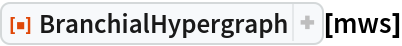Out=Use the resource function WolframModelPlot to visualize the branchial hypergraph:

 In:=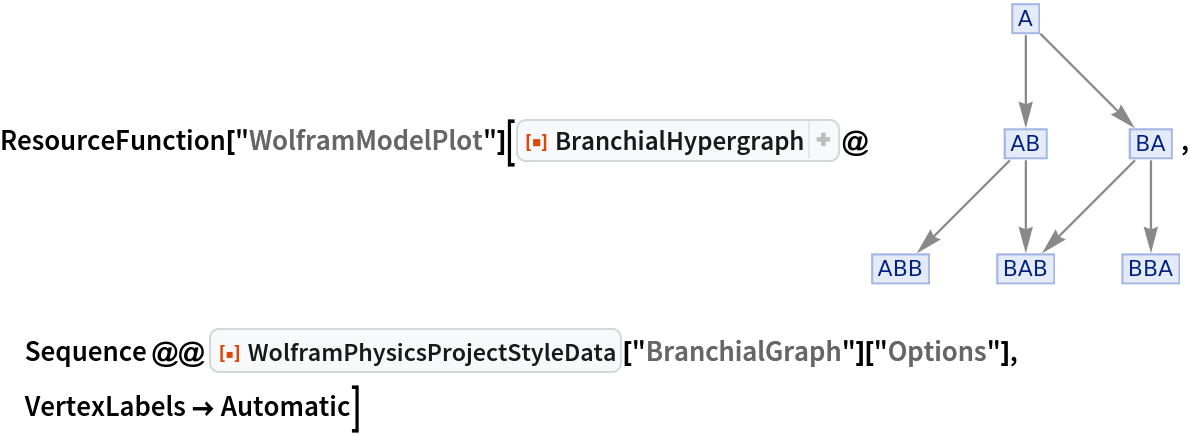Out=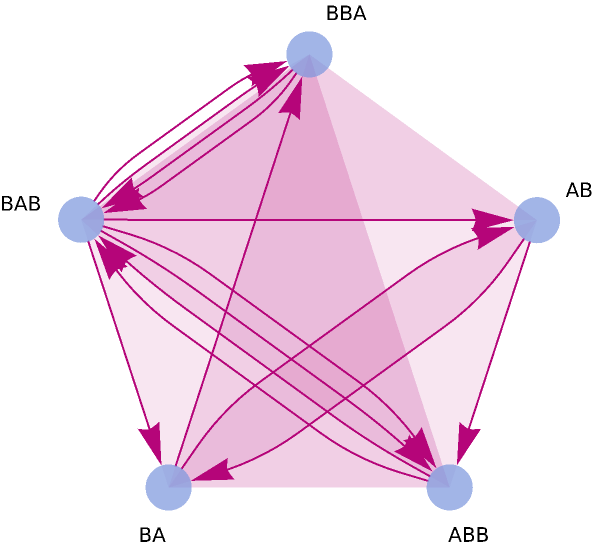### Options (1)

#### IncludeUnary (1)

Include unary hyperedges:

 In:=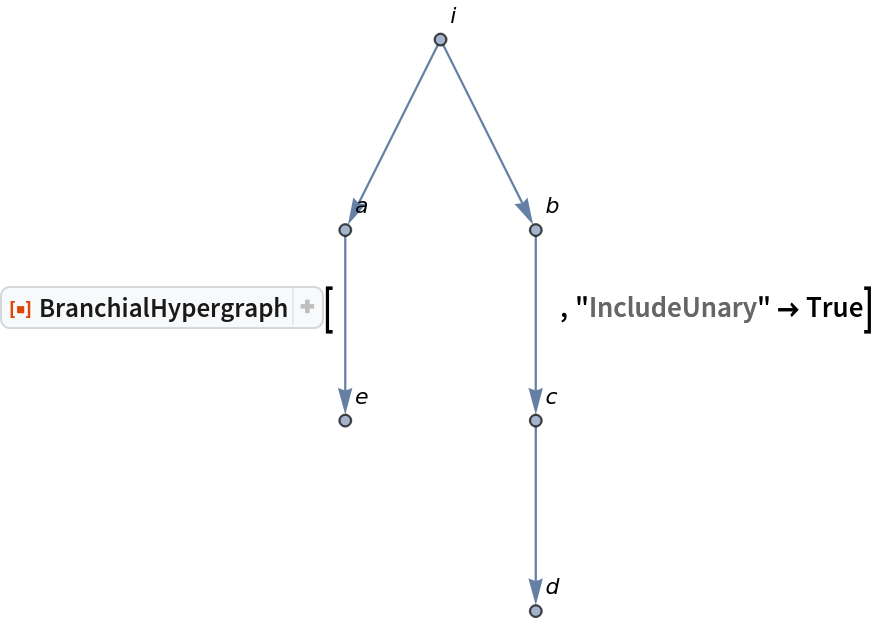Out=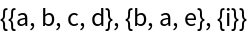### Properties and Relations (1)

Recover the branchial graph from the hypergraph:

 In:=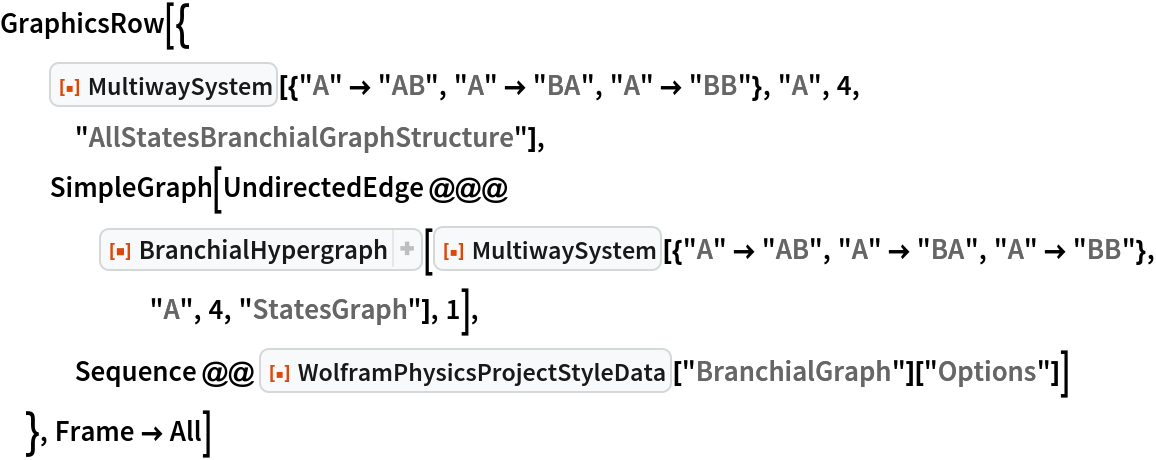Out=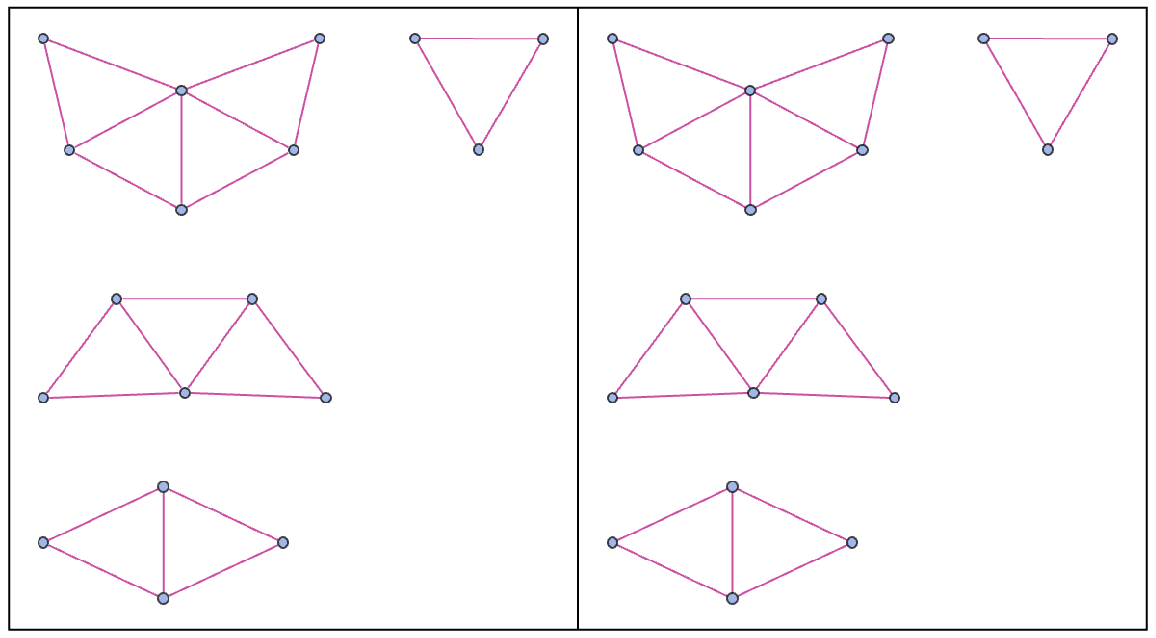N. Murzin

## Version History

• 1.1.0 – 08 November 2021
• 1.0.0 – 19 January 2021